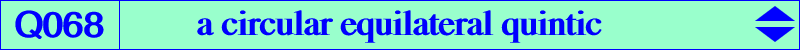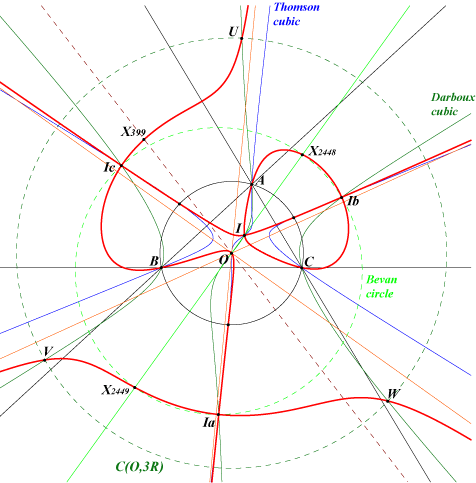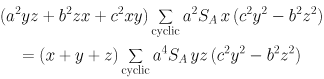X(1), X(3), X(164), X(399), X(2448), X(2449) excenters extraversions of X(164), X(2448), X(2449) common points of the circumcircle and the Thomson cubic infinite points of the McCay cubic three points U, V, W on C(O,3R) and on the Darboux cubicLet k be a real number, P a point and P* its isogonal conjugate. Consider the triangles : – P(k) homothetic of the pedal triangle of P under the homothety with center P, ratio k, – T(k) homothetic of ABC under the homothety with center P*, ratio k. These triangles P(k) and T(k) are orthologic and the centers of orthology are P and P*. They are perspective if and only if P lies on a circular quintic Q'(k). We have Q'(2) = Q067 and Q'(1) is the union of the circumcircle and the Darboux cubic. The limit case Q'(k->0) is Q068 and the limit case Q'(k->∞) is Q069. Q068 is a circular quintic with singular focus O. It has three real asymptotes parallel to those of the McCay cubic and also concurring at O. In other words, the polar lines of the five infinite points of Q068 pass through O. The locus of the finite points having a polar line with respect to Q068 passing through O is the Napoleon cubic K005. In particular, the tangents at A, B, C to Q068 pass through O. The common tangent at O to Q068 and K005 contains X(74), X(110), X(399), etc. The in/excenters are nodes with perpendicular nodal tangents. These tangents are parallel to the asymptotes of the rectangular circum-hyperbola passing through the corresponding in/excenter. These are the Feuerbach hyperbola for I and the Boutin hyperbolas for the excenters. K100 is the locus of points whose polar conic in Q068 is a rectangular hyperbola. In other words, the cubic is the Laplacian of the quintic. *** Q068 meets : – the circumcircle at the same points as the Thomson cubic. – the Bevan circle at the excenters and two points X(2448), X(2449) on the line OI. Under extraversion, we find six other points on the lines through O and the excenters. – the circle C(O, 3R) at three points U, V, W described in the Darboux cubic page and three other points on pK(X6, X631). – the circle C(O, 2R) at X(399) and three other points on pK(X6, X140). – more generally, the circle C(O, t R) (t is a positive real number) at six points, three of them on pK(X6, P1) and the three other on pK(X6, P2) where P1 and P2 are two points on the Euler line harmonically conjugated with respect to X(3) and X(5). These two points are given by OP1 = 1 / (2-t) OH and OP2 = 1 / (2+t) OH (vectors). *** Other locus properties • Q068 is the locus of P such that the generalized Lemoine cubic K(P) passes through the isogonal conjugate P* of P. • Let P be a point not lying on the line at infinity with pedal triangle A'B'C' and let Q be the isogonal conjugate of P. Denote by (Ca) the conic passing through A, P, Q, B', C' and define (Cb) and (Cc) similarly. (Ca), (Cb), (Cc) share the same tangent at P if and only if P lies on Q068. (Angel Montesdeoca, private message, 2018-02-11. See here).